• WACC - the forgotten cost of debt
Articles:

# WACC - the forgotten cost of debt

06 September 2021

In this post I want to touch on one of the most misapplied inputs I have observed when reviewing value in use (“VIU”) calculations for purpose of impairment testing in terms of IAS 36 — Impairment of Assets (“IAS 36”). Whilst relevant to valuations performed for IAS 36 impairment purposes, the observation equally applies to any discounted cash flow valuation of a business. IAS 36 requires that market inputs be used when valuing a cash-generating unit. When computing a Weighted Average Cost of Capital (“WACC”) the valuator assumes a target capital structure for purposes of calculating the WACC. This is usually determined with reference to capital structures observed for a publicly traded peer set, however one input that is often overlooked is how the target capital structure would affect the cost of debt.

Before delving further into capital structure, it is important to understand what the WACC represents. To put this simply, it is the weighted average return that investors (both debt and equity) require given the risk profile of an enterprise and the projected cash flows. The WACC is used as the discount rate to determine the present value of the expected cash flow of the firm to determine the enterprise value (“EV”). From a purely mathematical point of view, the higher the WACC the lower the EV, the lower the WACC the higher the EV, given two subject assets with the same forecast set of cash flows. Intuitively this makes sense, the market will pay less for more risky cash flows and more for less risky cash flows.

Consider the following, you have the option to invest in a South African government bond with a coupon of R1000 per annum or a new start-up which is forecasting to pay R1000 per annum to shareholders, but there is a high risk that the start-up will not actually make these payments and could fail completely. A rational investor will pay considerably more for the SA government bond which effectively guarantees R1000 per annum compared to a start-up that may or may not pay R1000 per annum.

As noted above the WACC is the weighted return to debt and equity investors in a firm. These investors have very different required returns as they have very different risk profiles.

Debt investors typically require a lower return (‘cost of debt’ or ‘𝐾𝑑’) on their investment compared to equity investors as they are exposed to lower risk. They receive their return before the equity holders and often have security over specific company assets, so even if the company cannot meet their debt payment obligations, debt investors are typically able to sell off a company’s assets to recover their capital.

Equity investors require a higher return (‘cost of equity’ or ‘𝐾𝑒’) as they are exposed to a higher level of risk. They have no contractual right to receive cash flows, the cash flows they do receive rank behind the debt holders and are only paid if the company has sufficient liquidity to do so, and if the company goes bankrupt, they will be last in line to share in the remaining asset value of the company and are unlikely to receive any portion of their capital back.

In calculating the WACC the 𝐾𝑑 is weighted by the total market value of debt relative to the enterprise value and the 𝐾𝑒 is weighted by the total market value of equity relative to the enterprise value, with the sum of these weighting being the WACC. This is expressed in an equation as follows: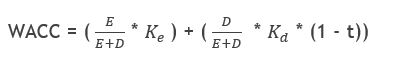where 𝐾𝑒 is the cost of equity, 𝐾𝑑 is the cost of debt, E is the market value of the firm’s equity, D is the market value of the firm’s debt and t is the corporate tax rate.

### Debt Equity Ratio

The assumption (and mistake) that is often made when performing an impairment test (or a valuation of a company) is that one can increase the weighting of the debt (cheaper financing) and decrease the weighting of equity (expensive financing), thereby decreasing the WACC and increasing the EV.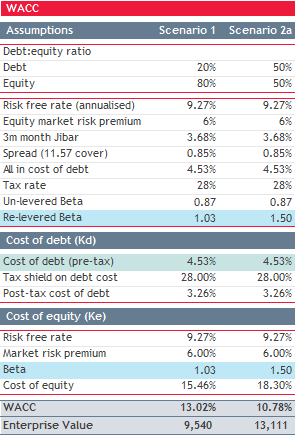The adjacent table illustrates the profound impact that adjusting the debt equity ratio has on the WACC and hence the valuation of a company (without appropriately accounting for the change in the cost of debt).

Under the 20% debt equity ratio scenario, and assuming constant pre-tax cash flows of R1000 escalating at 5% per annum the implied enterprise value is R9 540, whereas under the 50% debt equity scenario the implied enterprise value is R13 111, which is an increase of c.37% in value just by adjusting the debt equity ratio!

This begs the questions, can one increase the value of the company by 37% just by increasing the level of debt, and if this was the case why don’t companies simply increase debt to increase their value?

The answer is that while we have increased the cost of equity (through re-levering the beta), we have not considered the impact that the higher debt levels will have on the cost of debt. Unfortunately, this is where most analysis stops, resulting in the WACC being understated due to an understatement of the cost of debt and the company being overvalued.

Principally the increase in debt in a company will have two impacts (ignoring any impact of the tax deductibility of interest):

1. The higher leverage increases the risk to equity holders, and they will demand a higher rate of return, which has been addressed above; and
2. Due to the higher debt payment obligations (as there is implicitly more debt), the company will have less free cash flow to cover their debt obligations and debt holders will demand a higher return (typically via an increase in the spread charged to the firm by the lending institution) as there is a higher likelihood that the company will default on these payments (not accounted for above).

### Increase in the 𝐾𝑒

The increase in cost of equity is dealt with through the un-levering and re-levering the beta. Simply put, the lower the debt levels the lower the market beta, the lower the WACC, and the higher the debt levels, the higher the market beta, and the higher the WACC. In moving from Scenario 1 to Scenario 2a above, the cost of equity has increased from 15.46% to 18.3% due to the market beta increasing from 1.03 to 1.5.

In my experience, this adjustment is correctly dealt with in most WACC calculations.

Before exploring the debt component further, consider this question, what would happen to the cost of debt if the company was 100% debt funded?

1 I would argue that if the company was 100% debt funded the cost of debt would converge on the cost of equity.

### The cost of debt

Banks or other lending institutions typically quote debt either with refence to the South African Prime Rate or the Johannesburg Interbank Average Rate (“JIBAR”) which is modified by a spread they charge their client. Going forward I will only deal with JIBAR as JIBAR always has a positive spread, with a higher spread implying more risk .

In determining the spread the bank will run an internal credit model, which will assess the credit rating of the company. At the most fundamental level the bank is trying to assess the ability of the company to meet their obligations to the bank, being repayment of interest and capital, and the risk the company will default on the repayments. Therefore, while there are many complex credit models to assess this risk, calculating the companies interest cover ratio (“ICR”), being EBITDA divided by interest expense will provide a good indicative assessment of risk. For example, in almost all cases a company which has an ICR of 10 (i.e. their EBITDA can cover their interest expense 10 times) will be much less risky than a company with an ICR of 5.

For publicly traded debt, rating agencies such as S&P, Moody’s and Fitch will assess the risk of the traded debt and assign a risk rating, this is typically in the form of a symbol. In the case of S&P the least risky debt would be rated AAA+ and the lowest rated debt would be D.

So now that we have a basic understanding of how the banks and rating agencies assess the risk of lending to a company and hence the spread charged, let’s look at how we can incorporate the increased debt risk / cost of debt into the determination of WACC.

### Re-rating debt based on the ICR

Before we can consider adjusting the cost of debt, we need a way to equate an ICR to a spread. Thankfully, Aswath Damodaran, a professor of valuation at NYU Stern generates this data by regressing the ICR of publicly traded companies against the spread on their debt instruments.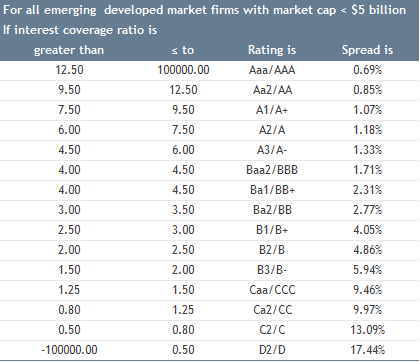Ideally one would want to determine this specifically for the South African market when valuing a South African company, however the universe of South African companies with traded debt instruments is small, and while the Damodaran regression will not give a perfect approximation it is better than not assessing the potential increase in risk at all. One should also consider calibrating this table to the company’s existing debt instruments.

2 Most small companies have their debt quoted relative to prime, i.e. prime less or plus a spread based on the risk profile. While the rates quoted to the company may be based on prime, the banks will be assessing this relative to an interbank rate (such as JIBAR) as this is their cost of capital.

3 BDO has utilized other methods of computing the cost of debt, such as asking the bank for indicative costing given a certain ICR (only really possible for clients with very strong relationships with their banks), or if the agreement quotes specific rates based on certain metrics, for example BDO worked on a client whose bank quoted them spreads based on their debt level relative to their EBITDA, and we utilized this to compute their new cost of debt.

For the debt component in the WACC from the above scenario, I calculated the initial spread using this table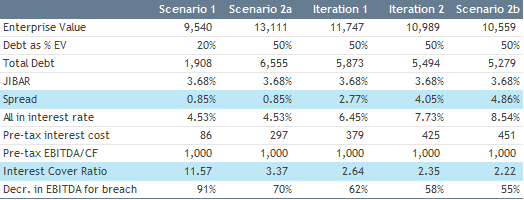In Scenario 1, I computed the cost of debt of 4.53% utilising the Damodaran table. I.e. based on the EBITDA of R1000 and an interest expense of R86 there is an interest cover ratio of 11.57, which implies a spread of 0.85%. This process is iterative which I will explain further in Scenario 2.

Under Scenario 2a with the new debt/ equity ratio without modifying the cost of debt, the EV increases to R13 111. Based on this EV the company will take on R6 555 of debt and have a pre-tax interest cost of R297, however this results in an of ICR 3.37 which implied a spread of 2.77% as opposed to 0.85%. We therefore iterate this process by increasing the spread in each iteration until the spread and the calculated ICR fall into the same bracket in the Damodaran table, i.e. in Scenario 2b our ICR is 2.22 which implied a spread of 4.86%, which both fall into the same bracket in the Damodaran table.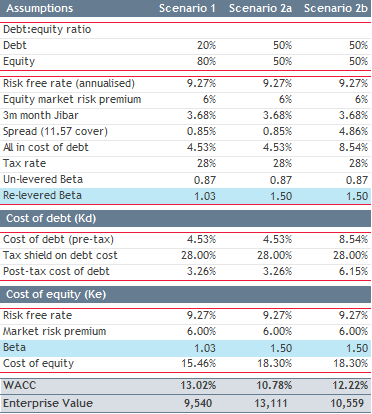While the process of re-rating the debt based on the ICR utilising the Damodaran regression analysis is not perfect, it provides a much more realistic view of a company’s WACC where the debt / equity ratio is modified.

Just to be clear I am not arguing that the WACC should be the same under all debt / equity scenarios, the optimization of a capital structure can increase the firm’s overall value, however it is important to assess the potential increases in the cost of debt, so as to not understate the WACC and overstate value which is what I have often observed in practice.

### Conclusion

When the capital structure of a company is aligned to either a market or even a management target capital structure for purposes of calculating the WACC, most practitioners (and other finance professionals) correctly deal with increases in the cost of equity (due to increased debt level) through un-levering and re-levering the industry beta. In my experience many do not consider the increased cost of debt associated with increased debt levels. This will necessarily result in an understatement of the WACC and an overstatement of the EV.

To counter this issue, it is important for a valuation practitioner to consider how the debt holders will respond to the increased debt levels and modify the cost of debt in the WACC to reflect this new reality. This can be done either through use of the data published by Damodaran, engaging with your clients existing financiers, or reviewing the existing debt instruments to see if the spread relative to an ICR (or other measure) is available.